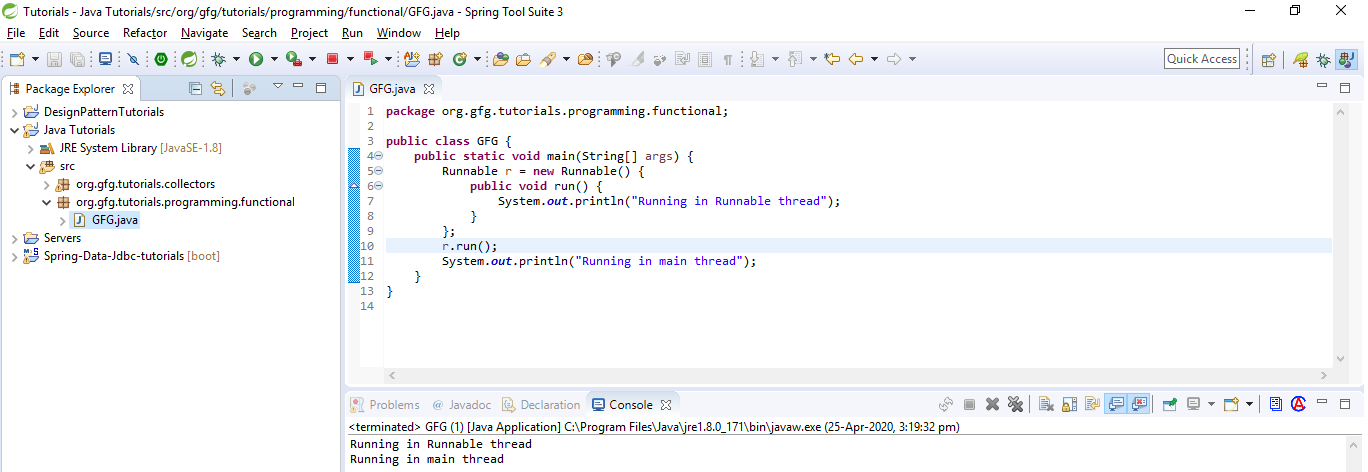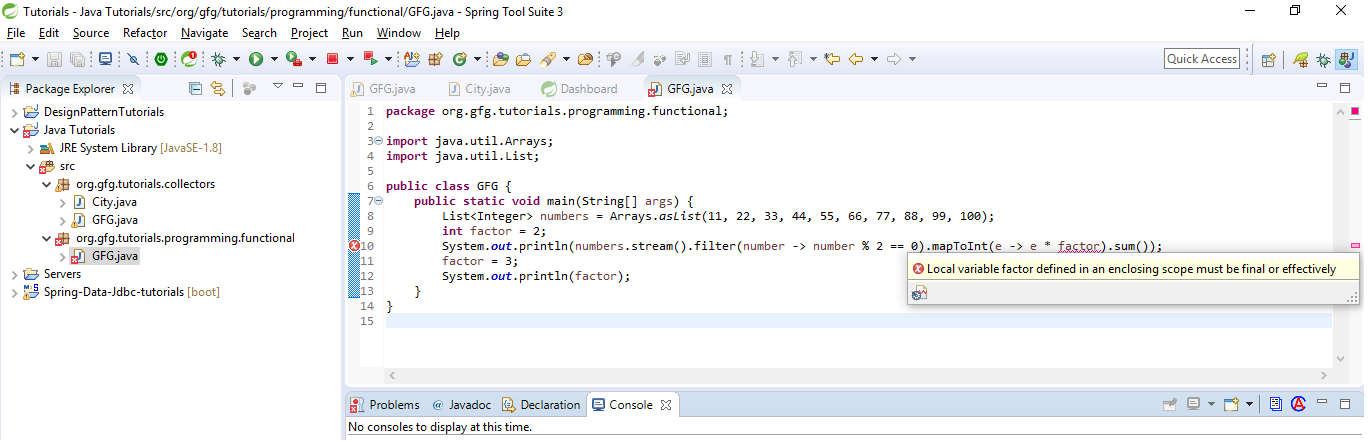Open In App

# Functional Programming in Java with Examples

So far Java was supporting the imperative style of programming and object-oriented style of programming. The next big thing what java has been added is that Java has started supporting the functional style of programming with its Java 8 release. In this article, we will discuss functional programming in Java 8.
What is functional programming?
It is a declarative style of programming rather than imperative. The basic objective of this style of programming is to make code more concise, less complex, more predictable, and easier to test compared to the legacy style of coding. Functional programming deals with certain key concepts such as pure function, immutable state, assignment-less programming etc.
Functional programming vs Purely Functional programming:
Pure functional programming languages don’t allow any mutability in its nature whereas a functional style language provides higher-order functions but often permits mutability at the risk of we failing to do the right things, which put a burden on us rather than protecting us. So, in general, we can say if a language provides higher-order function it is functional style language, and if a language goes to the extent of limiting mutability in addition to higher-order function then it becomes purely functional language. Java is a functional style language and the language like Haskell is a purely functional programming language.
Let’s understand a few concepts in functional programming

• Higher-order functions: In functional programming, functions are to be considered as first-class citizens. That is, so far in the legacy style of coding, we can do below stuff with objects.
1. We can pass objects to a function.
2. We can create objects within function.
3. We can return objects from a function.
4. We can pass a function to a function.
5. We can create a function within function.
6. We can return a function from a function.
• Pure functions: A function is called pure function if it always returns the same result for same argument values and it has no side effects like modifying an argument (or global variable) or outputting something.
• Lambda expressions: A Lambda expression is an anonymous method that has mutability at very minimum and it has only a parameter list and a body. The return type is always inferred based on the context. Also, make a note, Lambda expressions work in parallel with the functional interface. The syntax of a lambda expression is:

`(parameter) -> body`
• In its simple form, a lambda could be represented as a comma-separated list of parameters, the –> symbol and the body.

How to Implement Functional Programming in Java?

## java

 `// Java program to demonstrate``// anonymous method``import` `java.util.Arrays;``import` `java.util.List;``public` `class` `GFG {``    ``public` `static` `void` `main(String[] args)``    ``{` `        ``// Defining an anonymous method``        ``Runnable r = ``new` `Runnable() {``            ``public` `void` `run()``            ``{``                ``System.out.println(``                    ``"Running in Runnable thread"``);``            ``}``        ``};` `        ``r.run();``        ``System.out.println(``            ``"Running in main thread"``);``    ``}``}`

Output:

```Running in Runnable threadIf we look at run() methods, we wrapped it with Runnable. We were initializing this method in this way upto Java 7. The same program can be rewritten in Java 8 as:

## java

 `// Java 8 program to demonstrate``// a lambda expression``import` `java.util.Arrays;``import` `java.util.List;``public` `class` `GFG {``    ``public` `static` `void` `main(String[] args)``    ``{``        ``Runnable r``            ``= ()``            ``-> System.out.println(``                ``"Running in Runnable thread"``);` `        ``r.run();` `        ``System.out.println(``            ``"Running in main thread"``);``    ``}``}`

Output:

```Running in Runnable thread

Now, the above code has been converted into Lambda expressions rather than the anonymous method. Here we have evaluated a function that doesn’t have any name and that function is a lambda expression. So, in this case, we can see that a function has been evaluated and assigned to a runnable interface and here this function has been treated as the first-class citizen.
Refactoring some functions from Java 7 to Java 8:
We have worked many times with loops and iterator so far up to Java 7 as follows:

## java

 `// Java program to demonstrate an``// external iterator``import` `java.util.Arrays;``import` `java.util.List;``public` `class` `GFG {``    ``public` `static` `void` `main(String[] args)``    ``{``        ``List numbers``            ``= Arrays.asList(``11``, ``22``, ``33``, ``44``,``                            ``55``, ``66``, ``77``, ``88``,``                            ``99``, ``100``);` `        ``// External iterator, for Each loop``        ``for` `(Integer n : numbers) {``            ``System.out.print(n + ``" "``);``        ``}``    ``}``}`

Output:

`11 22 33 44 55 66 77 88 99 100`

Above was an example of forEach loop in Java a category of external iterator, below one is again example and another form of external iterator.

## java

 `// Java program to demonstrate an``// external iterator``import` `java.util.Arrays;``import` `java.util.List;``public` `class` `GFG {``    ``public` `static` `void` `main(String[] args)``    ``{``        ``List numbers``            ``= Arrays.asList(``11``, ``22``, ``33``, ``44``,``                            ``55``, ``66``, ``77``, ``88``,``                            ``99``, ``100``);` `        ``// External iterator``        ``for` `(``int` `i = ``0``; i < numbers.size(); i++) {``            ``System.out.print(numbers.get(i) + ``" "``);``        ``}``    ``}``}`

Output:

`11 22 33 44 55 66 77 88 99 100`

We can transform the above examples of an external iterator with an internal iterator introduced in Java 8, as follows:

## java

 `// Java 8 program to demonstrate``// an internal iterator` `import` `java.util.Arrays;``import` `java.util.List;` `public` `class` `GFG {``    ``public` `static` `void` `main(String[] args)``    ``{``        ``List numbers``            ``= Arrays.asList(``11``, ``22``, ``33``, ``44``,``                            ``55``, ``66``, ``77``, ``88``,``                            ``99``, ``100``);` `        ``// Internal iterator``        ``numbers.forEach(number``                        ``-> System.out.print(``                            ``number + ``" "``));``    ``}``}`

Output:

`11 22 33 44 55 66 77 88 99 100`

Here, the functional interface plays a major role. Wherever a single abstract method interface is expected, we can pass lambda expression very easily. Above code could be more simplified and improved as follows:

`numbers.forEach(System.out::println);`

Imperative Vs Declarative Programming:
The functional style of programming is declarative programming. In the imperative style of coding, we define what to do a task and how to do it. Whereas, in the declarative style of coding, we only specify what to do. Let’s understand this with an example. Given a list of number let’s find out the sum of double of even numbers from the list using an imperative and declarative style of coding.

## java

 `// Java program to find the sum``// using imperative style of coding``import` `java.util.Arrays;``import` `java.util.List;``public` `class` `GFG {``    ``public` `static` `void` `main(String[] args)``    ``{``        ``List numbers``            ``= Arrays.asList(``11``, ``22``, ``33``, ``44``,``                            ``55``, ``66``, ``77``, ``88``,``                            ``99``, ``100``);` `        ``int` `result = ``0``;``        ``for` `(Integer n : numbers) {``            ``if` `(n % ``2` `== ``0``) {``                ``result += n * ``2``;``            ``}``        ``}``        ``System.out.println(result);``    ``}``}`

Output:

`640`

The first issue with the above code is that we are mutating the variable result again and again. So mutability is one of the biggest issues in an imperative style of coding. The second issue with the imperative style is that we spend our effort telling not only what to do but also how to do the processing. Now let’s re-write above code in a declarative style.

## java

 `// Java program to find the sum``// using declarative style of coding``import` `java.util.Arrays;``import` `java.util.List;``public` `class` `GFG {``    ``public` `static` `void` `main(String[] args)``    ``{``        ``List numbers``            ``= Arrays.asList(``11``, ``22``, ``33``, ``44``,``                            ``55``, ``66``, ``77``, ``88``,``                            ``99``, ``100``);` `        ``System.out.println(``            ``numbers.stream()``                ``.filter(number -> number % ``2` `== ``0``)``                ``.mapToInt(e -> e * ``2``)``                ``.sum());``    ``}``}`

Output:

`640`

From the above code, we are not mutating any variable. Instead, we are transforming the data from one function to another. This is another difference between Imperative and Declarative. Not only this but also in the above code of declarative style, every function is a pure function and pure functions don’t have side effects.
In the above example, we are doubling the number with a factor 2, that is called Closure. Remember, lambdas are stateless and closure has immutable state. It means in any circumstances, the closure could not be mutable. Let’s understand it with an example. Here we will declare a variable factor and will use inside a function as below.

## java

 `// Java program to demonstrate an``// declarative style of coding``import` `java.util.Arrays;``import` `java.util.List;``public` `class` `GFG {``    ``public` `static` `void` `main(String[] args)``    ``{``        ``List numbers``            ``= Arrays.asList(``11``, ``22``, ``33``, ``44``,``                            ``55``, ``66``, ``77``, ``88``,``                            ``99``, ``100``);``        ``int` `factor = ``2``;``        ``System.out.println(``            ``numbers.stream()``                ``.filter(number -> number % ``2` `== ``0``)``                ``.mapToInt(e -> e * factor)``                ``.sum());``    ``}``}`

Output:

`640`

Above code works well, but now let’s try mutating it after its use and see what happens:

## java

 `import` `java.util.Arrays;``import` `java.util.List;``public` `class` `GFG {``    ``public` `static` `void` `main(String[] args)``    ``{``        ``List numbers``            ``= Arrays.asList(``11``, ``22``, ``33``, ``44``,``                            ``55``, ``66``, ``77``, ``88``,``                            ``99``, ``100``);``        ``int` `factor = ``2``;``        ``System.out.println(``            ``numbers.stream()``                ``.filter(number -> number % ``2` `== ``0``)``                ``.mapToInt(e -> e * factor)``                ``.sum());``          ``factor = ``3``;``    ``}``}`

The above code gives a compile-time error saying Local variable factor defined in an enclosing scope must be final or effectively final.

The time complexity of this program is O(n), where n is the number of elements in the list. This is because the program iterates over each element of the list once to apply the filter and map operations.

The space complexity of this program is O(1), because it only stores the input list and a few integers in memory, and the memory usage does not depend on the size of the input list.This means that here, the variable factor is by default being considered as final. In short, we should never try mutating any variable which is used inside pure functions. Doing so will violate pure functions rules which says pure function should neither change anything nor depend on anything that changes. Mutating any closure(here factor) is considered as a bad closure because closures are always immutable in nature.